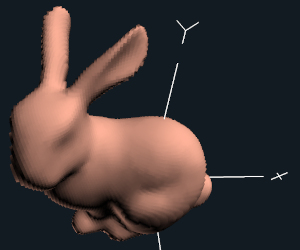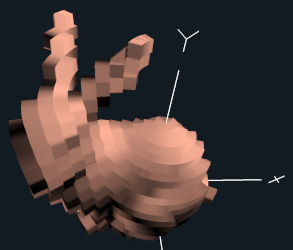## Project #7A

### Due: March 10

I will be making up an alternative Project #7 for those who do not have access to a system capable of doing Geometry Shaders.

### Introduction:

The word "quantize" means to take something that is continuous, and give it discrete (fixed) locations instead.

In this project, we are going to quantize an object in cyclindrical coordinates. You will add more detail to each triangle using the triangle interpolation (like in the sphere subdivision shader). You will then turn each x-y-z into a radius and height, quantize both quantities, then convert them back to x-y-z.

### Requirements:

1. Your input should be something with triangles, e.g., the teapot, the torus, an OBJ file, etc.

2. Have a uLevel slider that controls the number of levels of triangle subdivision.

3. Have a uQuantize variable that controls the quantization equation (see below).

4. Do some sort of lighting. The quick-and-dirty-diffuse is fine. Per-fragment would be even better.A Little Quantization A Lot of Quantization

### Hints

• A GLIB file could look something like this:
```##OpenGL GLIB
Perspective 70
LookAt  0 0 3     0 0 0    0 1 0

Vertex   cylquant.vert
Geometry cylquant.geom
Fragment cylquant.frag
Program  CylQuant  			\
uLevel <0 3 3>			\
uQuantize <1. 50. 50.>		\
uColor { 1.00 0.65 0.40 }

Obj bunny010n.obj
```

• Pass a vNormal from the vertex shader to the geometry shader.
```
out vec3	vNormal;

. . .

in vec3		vNormal;
```

• The geometry shader layouts will look like this:
```
layout( triangles )  in;
layout( triangle_strip, max_vertices=204 )  out;
```

• The geometry shader must set gl_Position and all out variables before each call to EmitVertex( ).

• If you are doing a quick-and-dirty diffuse lighting, pass a gLightIntensity from the geometry shader to the rasterizer to the fragment shader.
```
out float	gLightIntensity;

. . .

in float	gLightIntensity;
```

• If you are using the better per-fragment lighting, pass a gNs, gEs, gLs from the geometry shader to the rasterizer to the fragment shader.
```
out vec3	gNs, gEs, gLs;

. . .

in vec3		gNs, gEs, gLs;
```

• Use uLevel to subdivide the triangle into smaller triangles. Use the s and t interpolation scheme from our class notes:
```
vec3 v = V0 + s*V01 + t*V02;
```
where we created new (x,y,z)'s to form triangle strips. You can use the code from the Sphere Subdivision geometry shader as a start.

Use the same equation to also create new (nx,ny,nz)'s:

```
vec3 n = N0 + s*N01 + t*N02;
```
and then multiply by gl_NormalMatrix.

• You can quantize a single float like this:
```float
Sign( float f )
{
if( f >= 0. )   return  1.;
return -1.;
}

float
Quantize( float f )
{
f *= uQuantize;
f += 0.5 * Sign(f);                // round-off
int fi = int( f );
f = float( fi ) / uQuantize;
return f;
}
```

• This is a good way to handle arctangents in GLSL:
```const float PI = 3.14159265;

float
atan2( float y, float x )
{
if( x == 0. )
{
if( y >= 0. )
return  PI/2.;
else
return -PI/2.;
}
return atan(y,x);
}
```

• To turn a Cartesian v = vec3(x,y,z) into cylindrical coordinates (radius,height), you do this:
```float radius = length( v.xz );
float theta = atan2( v.z, v.x );
float height = v.y;
```

Leave the angle theta alone, and quantize both radius and height;

• To turn a cylindrical coordinate (radius,height) back into a Cartesian v = vec3(x,y,z) for drawing, you do this:
```v.xz = radius * vec2( cos(theta), sin(theta) );
v.y = height;
```

• Don't do any matrix multiplications in the vertex shader, just do gl_Position = gl_Vertex with no modification.

• In the geometry shader, the overall flow could be something like:
```
V0, V1, and V2 are the corner points of the original triangle.
V01 = V1 - V0
V02 = V2 - V0

N0, N1, and N2 are the corner normals of the original triangle.
N01 = N1 - N0
N02 = N2 - N0

for each new triangle created by the triangle subdivision:
{
for each (s,t) corner point in that new triangle:
{
Turn that (s,t) into an (nx,ny,nz) using the triangle interpolation equation
Transform and normalize that (nx,ny,nz)
Use the (nx,ny,nz) to produce gLightIntensity

Turn that same (s,t) into an (x,y,z) using the triangle interpolation equation
Quantize radius and height, leaving theta alone
Turn the quantized (radius,height) back into (x,y,z)
Multiply the resulting (x,y,z) by the ModelviewProjection matrix to produce gl_Position
EmitVertex( );
}
}
```

• Don't forget that you still need to signify the end of the triangle strips with EndPrimitive( ).

• When you quantize coordinates like this, many elements of the geometry end up in the same place. Z-fighting is to be expected.

### The Turn-In Process:

Your turnin will be done at http://teach.engr.oregonstate.edu and will consist of:
1. All source files (.cpp, .glib, .vert, .geom, .frag). You can zip this all together if you want.
3. Be sure your video's protection is set to unlisted.
4. Do not put your PDF into your zip file. Leave it out separately so my collect-all-the-PDFs script can find it.

Be sure that your PDF file is turned-in separately (not part of a .zip file).